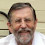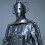### Kinematics of the stellar halo and the mass distribution of the Milky Way using BHB stars

I've been back for about a week, but it has been busy with a number of things.

Firstly, success in the current round Australian Research Council Discovery Projects. I'll write about this in a little more detail soon, but here's the summary.
There has been some uncertainty in the current round of funding, but it has all come out in the wash.

But there is more news. When I was traveling, PhD student, Parjwal Kafle, had his paper on measuring the mass distribution in the Milky Way.

This might strike you as a little odd. We live in the Milky Way, and it is the most studied galaxy in the entire Universe. Surely we know the mass of our own home galaxy? Don't we just add up all the stars we can see?

Well, if the stars were all there is, that would be correct. But we know there is more, much much more! There is dark matter, this stuff that dominates the gravitational attraction of the Universe. Our Sun is kept in its orbit by the halo of dark matter that surrounds the Galaxy.
Now we can see the problem. As well as there being a lot of dark matter, it is also more distributed than the stars we can see.

Now, normally we use "kinematic tracers" (fancy words for the speeds of stars) to measure the amount of mass present (including dark matter), but out there in the halo, where there is a lot of mass, there doesn't appear to be any stars.

But, there are a smattering of stars out there, in what is known as the stellar halo, although they are far away, and so we can really see only the brightest ones.

There are still a couple of problems. The density of these halo stars on the sky is generally quite low, and there are a lot of "contaminants" - annoying stars nearby in the Galaxy that look like they are far away in the halo.

The secret is to look at a large amount of the sky, and in recent years we've gotten sensitive telescopes to do this. with one of the best being the Sloan Digital Sky Survey. I've not got a enough time to go through the details, but this program identified distant halo stars, namely stars on the Blue Horizontal Branch.

But this is just the data. How do you measure the mass? This is a tricky problem as we don't have that many tracers, and while we know the positions on the sky well, but the distances can be a uncertain, and while we might have the velocity of the star along the line of sight, we don't know its full 3d velocity.

The answer is not straight-forward, but essentially to have to try and generate a model of mass distribution of mass and stars and see if it matches the properties of stars on the sky.  In summary, this takes a lot of calculations (lots of Bayesian modeling). But what's the result? The mass of the Galaxy is found to be
This is a cool result! If you are not in the field, you may look at the uncertainties (the +0.5 and -0.4 in there) and say "You don't know this better than about 30%". But this is the state of play. This is how well we understand the amount of mass in the Milky Way.

To illustrate this, here's a picture of the rotation curve of the that Prajwal works out.
The red line is Prajwal's fit, but look at the data! Look at how noisy it is. Clearly, we still have a lot to learn about our Milky Way galaxy. We're working on it :)

Well done Prajwal!

Kinematics of the stellar halo and the mass distribution of the Milky Way using BHB stars

Prajwal R. Kafle, Sanjib Sharma, Geraint F. Lewis, Joss Bland-Hawthorn
Here we present a kinematic study of the Galactic halo out to a radius of $\sim$ 60 kpc, using 4664 blue horizontal branch (BHB) stars selected from the SDSS/SEGUE survey, to determine key dynamical properties. Using a maximum likelihood analysis, we determine the velocity dispersion profiles in spherical coordinates ($\sigma_{r}$, $\sigma_{\theta}$, $\sigma_{\phi}$) and the anisotropy profile ($\beta$). The radial velocity dispersion profile ($\sigma_{r}$) is measured out to a galactocentric radius of $r \sim 60$ kpc, but due to the lack of proper-motion information, $\sigma_{\theta}$, $\sigma_{\phi}$ and $\beta$ could only be derived directly out to $r \sim25$ kpc. From a starting value of $\beta\approx 0.5$ in the inner parts ($9<r/\kpc<12$), the profile falls sharply in the range $r \approx 13-18$ kpc, with a minimum value of $\beta=-1.2$ at $r=17$ kpc, rising sharply at larger radius. In the outer parts, in the range $25<r/\kpc<56$, we predict the profile to be roughly constant with a value of $\beta\approx 0.5$. The newly discovered kinematic anomalies are shown not to arise from halo substructures. We also studied the anisotropy profile of simulated stellar halos formed purely by accretion and found that they cannot reproduce the sharp dip seen in the data. From the Jeans equation, we compute the stellar rotation curve ($v_{\rm circ}$) of the Galaxy out to $r \sim 25$ kpc. The mass of the Galaxy within $r \lesssim 25$ kpc is determined to be $2.1 \times 10^{11}$ $M_{\sun}$, and with a 3-component fit to $v_{\rm circ}(r)$, we determine the virial mass of the Milky Way dark matter halo to be $M_{\rm vir} = 0.9 ^{+0.4}_{-0.3} \times 10^{12}$ $M_{\sun}$ ($R_{\rm vir} = 249^{+34}_{-31}$ kpc).

1.Is there any particular reason why you chose BHB stars to detect the Milky Way Halo survey? I recall you telling us that you used RGB stars in the PAndAS survey to detect the M33/M31 halo?

Are both types "standard candles"?

2.3 consecutive posts on the halo (M33, M31 and now MW) show how important studies of the halo is to disentangle formation history of the Galaxy.

3.Why BHB? The first thing is that they are bright, so we can see them a long way out into the halo. The big problem is confusion of the bright distant things with the fainter nearby things, and so a lot of work is done to try and generate a clean catalog of the distant things. Luckily for us, someone has worked out how to do this for BHB stars which we use.

4.Thanks Geraint. Your posts on research items like this always inspire me to try and find out a little more basic info about the topic.

I frequently do look at the published papers as you suggested but unfortunately, they are by necessity not couched in laymens language, so nine times out of ten I pick up very little from them. They also tend to start where other papers have finished, so the big picture is not visible.

5.Yes - papers are written for those that already know the jargon etc. I'm sure the SDSS people give public talks, but I must admit that I don't know if there is a place that they store the talks, although there are a few things here

http://www.sdss.org/education/

If you have questions, let me know. If you would like my take on a topic, let me know and I'll see if I can prepare a blog post :)